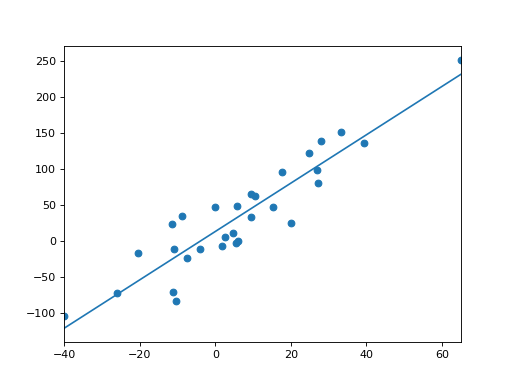# statsmodels.graphics.regressionplots.abline_plot¶

statsmodels.graphics.regressionplots.abline_plot(intercept=None, slope=None, horiz=None, vert=None, model_results=None, ax=None, **kwargs)[source]

Plots a line given an intercept and slope.

interceptfloat

The intercept of the line

slopefloat

The slope of the line

horizfloat or array-like

Data for horizontal lines on the y-axis

vertarray-like

Data for verterical lines on the x-axis

model_resultsstatsmodels results instance

Any object that has a two-value params attribute. Assumed that it is (intercept, slope)

axaxes, optional

Matplotlib axes instance

kwargs

Options passed to matplotlib.pyplot.plt

Returns
figFigure

The figure given by ax.figure or a new instance.

Examples

>>> import numpy as np
>>> import statsmodels.api as sm

>>> np.random.seed(12345)
>>> X = sm.add_constant(np.random.normal(0, 20, size=30))
>>> y = np.dot(X, [25, 3.5]) + np.random.normal(0, 30, size=30)
>>> mod = sm.OLS(y,X).fit()
>>> fig = sm.graphics.abline_plot(model_results=mod)
>>> ax = fig.axes
>>> ax.scatter(X[:,1], y)
>>> ax.margins(.1)
>>> import matplotlib.pyplot as plt
>>> plt.show()